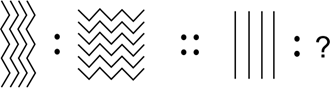﻿ Reasoning Olympiad (CRO) Sample Paper & Questions for Class 1

# CREST Reasoning Olympiad Class 1 Sample Paper

Sample PDF of CREST Reasoning Olympiad for Class 1:

## Syllabus:

Section 1: Analogy, Geometrical Shapes, Grouping of Figures, Embedded Figures, Odd One Out, Pattern, Measuring Units, Ranking Test, Spatial Understanding.

Achievers Section: Higher Order Thinking Questions - Syllabus as per Section 1

 Q.1 There is a certain relationship between the pair of figures given on either side of (::). Identify the relationship of the given pair and find the matching term.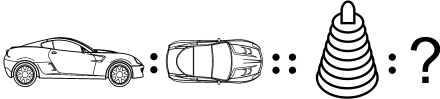Q.2 Which group does figure X belong to?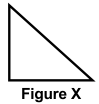Q.3 Given below is an object. Which among the following matches with the given object?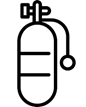Q.4 Which among the following will you select to complete the pattern?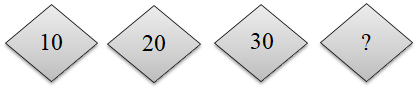Q.5 Find the odd one out from the given options:
 Q.6 Find the odd one out from the given options:
 Q.7 If there are 11 students in a group project and your position is 6th from the start, then what is your position from the last?
 Q.8 There is a certain relationship between the pair of figures given on either side of (::). Identify the relationship of given pair and find the matching term.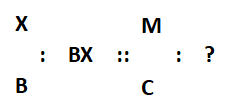Q.9 What is the position of the pumpkin from the right?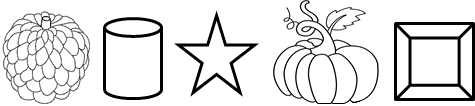Q.10 There is a certain relationship between the pair of figures given on either side of (::) identify the relationship of given pair and find the matching term.